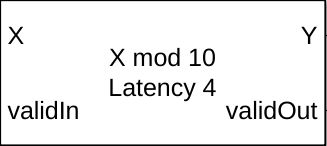# Modulo by Constant HDL Optimized

Perform mod operation with a constant denominator and generate optimized HDL code

Since R2021a

•Libraries:
Fixed-Point Designer HDL Support / Math Operations

## Description

The Modulo by Constant HDL Optimized block performs the modulo operation (remainder after division) with a constant denominator using an HDL-optimized architecture with cycle-true latency.

The Modulo by Constant block uses an algorithm that is functionally similar to a Barrett Reduction. The division operation is computed via a multiplication by inverse, which generally results in better performance on embedded systems.

## Ports

### Input

expand all

Dividend, specified as a real scalar.

If X is a fixed-point data type, it must use binary-point scaling. Slope-bias representation is not supported for fixed-point data types.

Data Types: `single` | `double` | `int8` | `int16` | `int32` | `int64` | `uint8` | `uint16` | `uint32` | `fixed point`

Whether input is valid, specified as a Boolean scalar. This control signal indicates when the data from the X input port is valid. When this value is `1` (`true`), the block captures the value on the X input port. When this value is `0` (`false`), the block ignores the input samples.

Data Types: `Boolean`

### Output

expand all

Result of modulus operation, returned as a scalar.

Data Types: `single` | `double` | `int8` | `int16` | `int32` | `int64` | `uint8` | `uint16` | `uint32` | `fixed point`

Whether the output data is valid, returned as a Boolean scalar. When the value of this control signal is `1` (`true`), the block has successfully computed the output Y. When this value is `0` (`false`), the output data is not valid.

Data Types: `Boolean`

## Parameters

expand all

Divisor to use for the modulus operation, specified as a positive, real-valued, finite scalar.

#### Programmatic Use

 Block Parameter: `Denominator` Type: character vector Values: MATLAB® expression that evaluates to a positive, real-valued, finite fixed point or numeric value Data Types: `single` | `double` | `int8` | `int16` | `int32` | `int64` | `uint8` | `uint16` | `uint32` | `uint64` | `Boolean` | ```fixed point``` Default: `'10'`

## Algorithms

The Modulo by Constant HDL Optimized block performs the modulo operation (remainder after division) with a constant denominator using an HDL-optimized architecture with cycle-true latency.

The modulo operation,

`$Y=X\mathrm{mod}D=X-⌊\frac{X}{D}⌋×D$`

is an important building block for many mathematical algorithms. However, this formula for X mod D is computationally inefficient for fixed-point and integer inputs. Many embedded processors lack instructions for integer division. Those that do have them require many clock cycles to compute the answer. Division is also inefficient in commercially-available FPGAs, whose arithmetic circuits are designed for efficient multiplication, addition, and subtraction. Finally, for fixed-point modulo operations, it is difficult to optimize the word length of internal data types used for the calculation because the division operation is unbounded, even for small-wordlength inputs.

The denominator in the modulo problem is a compile-time constant, so the block can compute the floored division by using a multiplication followed by a cast. Rewriting the division operation as

`$\frac{X}{D}=X×\frac{1}{D}$`

shows this. The constant is calculated to the precision necessary to maintain both accuracy and computational efficiency. The cast that follows discards any fractional bits, which is an efficient operation on both microprocessors and FPGAs.

The Modulo by Constant block uses an algorithm that is functionally similar to a Barrett Reduction. The division operation is computed via a multiplication by inverse, which generally results in better performance on embedded systems.

## Version History

Introduced in R2021a